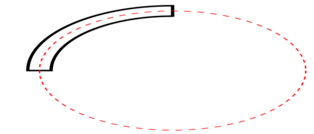Arcs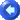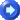Arcs make great colorbands on gauges.  They are versatile and easy to work with.  Like many other objects, arcs use a brush and pen.

The arc can be edited in the properties window with six different values:

PROPERTY REFERENCE

Center Point

This represents where you want the center of the arc to be located.  The center point for an arc is the point from which the radii will be calculated (see below).  To select a center point, you can either enter in the coordinates (see coordinate system for more information about these coordinates) or you can click the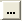box (which appears once you click inside where you would enter coordinates).  When you click this box, you can set your mouse on the point you would like and click.

This length refers to the distance from the center point to the edge of the arc (horizontally).

The length of the radius is a percentage of the window.  The radius is the length from the center point to the arc.  Therefore, the length of the entire arc is twice the length of the radius (from one edge to the center point to the other edge).  If, for example, you would like your arc to be half the length of the application window, set your horizontal radius to 25.  This means that from one edge of the ellipse to the center is 25%, or 1/4, of the screen.  So from one edge to the other it will be 50%, or half, of the screen.

This length refers to the distance from the center point to the edge of the arc (vertically). The same rules apply to this radius as the horizontal radius, which is explained above.

Arc Angle

 This is where you select where you would like the arc to start.   The image to the right shows the degrees along the circle.  If you would like the arc to start at the leftmost point, for example, enter in 180 for the arc angle.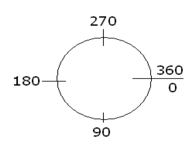Arc Sweep

This refers to how long you want the arc to be.  90 is 1/4 of an ellipse.  So if you want an arc that is 1/2 of an ellipse, you would enter 180.  If you want a smaller arc that is 1/8 of an ellipse you would enter 45.  Click here for more information on angles and degrees.

Arc Width

 This is the value you would adjust to increase or decrease the width of the arc.  Half of the width is distributed to each side of the ellipse that would have been drawn using the same radii and center point.